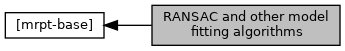Main MRPT website > C++ reference for MRPT 1.5.7
RANSAC and other model fitting algorithms

## Detailed Description

Collaboration diagram for RANSAC and other model fitting algorithms:## Classes

class  mrpt::math::ModelSearch
Model search implementations: RANSAC and genetic algorithm. More...

class  mrpt::math::RANSAC_Template< NUMTYPE >
A generic RANSAC implementation with models as matrices. More...

## Typedefs

typedef RANSAC_Template< double > mrpt::math::RANSAC
The default instance of RANSAC, for double type. More...

## RANSAC detectors

template<typename NUMTYPE >
void BASE_IMPEXP mrpt::math::ransac_detect_3D_planes (const Eigen::Matrix< NUMTYPE, Eigen::Dynamic, 1 > &x, const Eigen::Matrix< NUMTYPE, Eigen::Dynamic, 1 > &y, const Eigen::Matrix< NUMTYPE, Eigen::Dynamic, 1 > &z, std::vector< std::pair< size_t, TPlane > > &out_detected_planes, const double threshold, const size_t min_inliers_for_valid_plane=10)
Fit a number of 3-D planes to a given point cloud, automatically determining the number of existing planes by means of the provided threshold and minimum number of supporting inliers. More...

template<typename NUMTYPE >
void BASE_IMPEXP mrpt::math::ransac_detect_2D_lines (const Eigen::Matrix< NUMTYPE, Eigen::Dynamic, 1 > &x, const Eigen::Matrix< NUMTYPE, Eigen::Dynamic, 1 > &y, std::vector< std::pair< size_t, TLine2D > > &out_detected_lines, const double threshold, const size_t min_inliers_for_valid_line=5)
Fit a number of 2-D lines to a given point cloud, automatically determining the number of existing lines by means of the provided threshold and minimum number of supporting inliers. More...

template<class POINTSMAP >
void mrpt::math::ransac_detect_3D_planes (const POINTSMAP *points_map, std::vector< std::pair< size_t, TPlane > > &out_detected_planes, const double threshold, const size_t min_inliers_for_valid_plane)
A stub for ransac_detect_3D_planes() with the points given as a mrpt::maps::CPointsMap. More...

## ◆ RANSAC

 typedef RANSAC_Template mrpt::math::RANSAC

The default instance of RANSAC, for double type.

Definition at line 81 of file ransac.h.

## ◆ ransac_detect_2D_lines()

template<typename NUMTYPE >
 void BASE_IMPEXP mrpt::math::ransac_detect_2D_lines ( const Eigen::Matrix< NUMTYPE, Eigen::Dynamic, 1 > & x, const Eigen::Matrix< NUMTYPE, Eigen::Dynamic, 1 > & y, std::vector< std::pair< size_t, TLine2D > > & out_detected_lines, const double threshold, const size_t min_inliers_for_valid_line = `5` )

Fit a number of 2-D lines to a given point cloud, automatically determining the number of existing lines by means of the provided threshold and minimum number of supporting inliers.

Parameters
 out_detected_lines The output list of pairs: number of supporting inliers, detected line. threshold The maximum distance between a point and a temptative line such as the point is considered an inlier. min_inliers_for_valid_line The minimum number of supporting inliers to consider a line as valid.

## ◆ ransac_detect_3D_planes() [1/2]

template<typename NUMTYPE >
 void BASE_IMPEXP mrpt::math::ransac_detect_3D_planes ( const Eigen::Matrix< NUMTYPE, Eigen::Dynamic, 1 > & x, const Eigen::Matrix< NUMTYPE, Eigen::Dynamic, 1 > & y, const Eigen::Matrix< NUMTYPE, Eigen::Dynamic, 1 > & z, std::vector< std::pair< size_t, TPlane > > & out_detected_planes, const double threshold, const size_t min_inliers_for_valid_plane = `10` )

Fit a number of 3-D planes to a given point cloud, automatically determining the number of existing planes by means of the provided threshold and minimum number of supporting inliers.

Parameters
 out_detected_planes The output list of pairs: number of supporting inliers, detected plane. threshold The maximum distance between a point and a temptative plane such as the point is considered an inlier. min_inliers_for_valid_plane The minimum number of supporting inliers to consider a plane as valid.

Referenced by mrpt::math::ransac_detect_3D_planes().

## ◆ ransac_detect_3D_planes() [2/2]

template<class POINTSMAP >
 void mrpt::math::ransac_detect_3D_planes ( const POINTSMAP * points_map, std::vector< std::pair< size_t, TPlane > > & out_detected_planes, const double threshold, const size_t min_inliers_for_valid_plane )
inline

A stub for ransac_detect_3D_planes() with the points given as a mrpt::maps::CPointsMap.

Definition at line 61 of file ransac_applications.h.

References mrpt::math::ransac_detect_3D_planes().

 Page generated by Doxygen 1.8.14 for MRPT 1.5.7 Git: 8277875f6 Mon Jun 11 02:47:32 2018 +0200 at lun oct 28 01:50:49 CET 2019